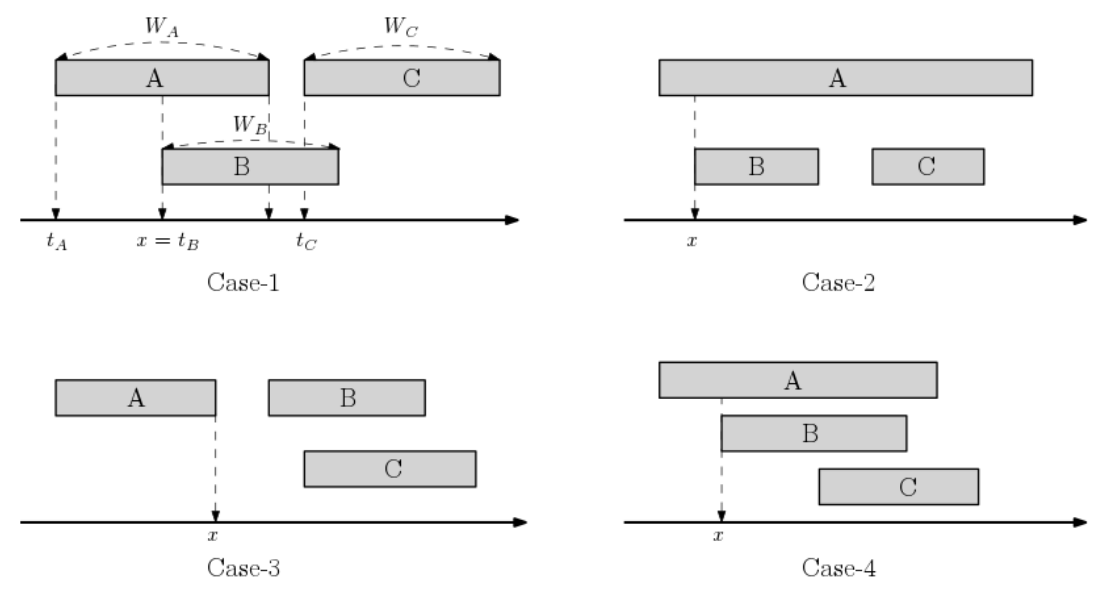시간 제한 메모리 제한 제출 정답 맞은 사람 정답 비율
1 초 512 MB 35 17 17 58.621%

문제

Three secret agents A, B and C want to confirm if their secret codes are identical. To keep this secret, they did not set the meeting time. Instead, they decided to appear randomly at a café during the time interval [0, S] of a day. Let tA, tB, tC denote the arrival time of A, B, and C to the café, respectively. So, tA, tB, tC are random numbers chosen uniformly from time interval [0, S].

The code confirmation proceeds as follows. The agent who arrives earlier waits for the next agents to appear for a predetermined waiting time. If two agents encounter in the café, both check if their codes are identical. Then the agent who arrived earlier leaves the café immediately after the code confirmation. The second agent then waits till the third agent appears at the café. The agent leaves the café if the agent encounters the last agent in the agent’s own waiting time.

The waiting times of three agents A, B, C have already been determined as wA, wB, wC. It is crucial that each of the arrival times tA, tB, tC is a real number between 0 and S, not necessarily an integer, whereas each of the waiting times wA, wB, wC is a positive integer satisfying 0 < wA + wB, wB + wC, wA + wC < S. We assume it takes no time in code confirmation task. The agent immediately leaves the café if the agent confirms the code with the agent arrived later. Let us explain this procedure using Figure G.1.Figure G.1 Four cases for successful and unsuccessful confirmations.

Successful code confirmation needs at least two encounters among three agents. When the arrival order is A, B, C, both of Case-1 and Case-4 are examples for successful confirmation, but Case-2 and Case-3 are not. In Case-1, the agent A leaves the café at time x = tB immediately not waiting by time tA + wA. It is easy to see that Case-2 is an unsuccessful case. In Case-2, though the waiting time of A overlaps those of B and C, B cannot confirm the code with C since the agent A (who already confirmed the code with B) leaves the café at time x. So, there is no way to confirm the code between B and C. Note that A also leaves the café at time x in Case-3 and Case-4.

We know the probability of the successful code confirmation depends on four integers (S, wA, wB, wC). We are given n different scenarios determined by four integers (S, wA, wB, wC). We want to sort these n scenarios in terms of this confirmation probability.

Given n scenarios, write a program to print the scenario indices in the non-decreasing order of the probability.

입력

Your program is to read from standard input. The input starts with a line containing an integer n (3 ≤ n ≤ 20), where n is the number of scenarios. In the following n lines, each line contains four positive integers S, wA, wB, wC in this order, describing a scenario (S, wA, wB, wC) where 0 < wA + wB, wB + wC, wA + wC ≤ 1,000.

출력

Your program is to write to standard output. Print the indices of n scenarios in the non-decreasing order of the probability in one line. If scenarios i and j for 1 ≤ i < jn have the same probability, then print i before j.

3
100 12 13 14
110  8  9 15
200 23 30 40

2 1 3

6
201 15 16 16
375 30 32 27
900 75 73 67
203 16 17 16
373 31 32 27
895 73 75 66

1 2 3 6 5 4